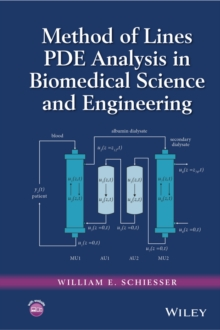Supporting your high street Find out how »
• My AccountBasket# Method of Lines PDE Analysis in Biomedical Science and Engineering Hardback

#### Description

Presents the methodology and applications of ODE and PDE models within biomedical science and engineering With an emphasis on the method of lines (MOL) for partial differential equation (PDE) numerical integration, Method of Lines PDE Analysis in Biomedical Science and Engineering demonstrates the use of numerical methods for the computer solution of PDEs as applied to biomedical science and engineering (BMSE).

Written by a well-known researcher in the field, the book provides an introduction to basic numerical methods for initial/boundary value PDEs before moving on to specific BMSE applications of PDEs.

Featuring a straightforward approach, the book s chapters follow a consistent and comprehensive format.

First, each chapter begins by presenting the model as an ordinary differential equation (ODE)/PDE system, including the initial and boundary conditions. Next, the programming of the model equations is introduced through a series of R routines that primarily implement MOL for PDEs.

Subsequently, the resulting numerical and graphical solution is discussed and interpreted with respect to the model equations. Finally, each chapter concludes with a review of the numerical algorithm performance, general observations and results, and possible extensions of the model. Method of Lines PDE Analysis in Biomedical Science and Engineering also includes: * Examples of MOL analysis of PDEs, including BMSE applications in wave front resolution in chromatography, VEGF angiogenesis, thermographic tumor location, blood-tissue transport, two fluid and membrane mass transfer, artificial liver support system, cross diffusion epidemiology, oncolytic virotherapy, tumor cell density in glioblastomas, and variable grids * Discussions on the use of R software, which facilitates immediate solutions to differential equation problems without having to first learn the basic concepts of numerical analysis for PDEs and the programming of PDE algorithms * A companion website that provides source code for the R routines Method of Lines PDE Analysis in Biomedical Science and Engineering is an introductory reference for researchers, scientists, clinicians, medical researchers, mathematicians, statisticians, chemical engineers, epidemiologists, and pharmacokineticists as well as anyone interested in clinical applications and the interpretation of experimental data with differential equation models. The book is also an ideal textbook for graduate-level courses in applied mathematics, BMSE, biology, biophysics, biochemistry, medicine, and engineering.

#### Information

£90.95

£76.39

on all orders

###### Pick up orders

from local bookshops

£138.00

£131.10

£109.99

£59.95

£79.95

£79.25